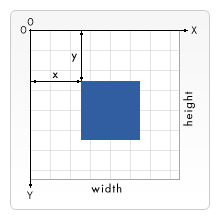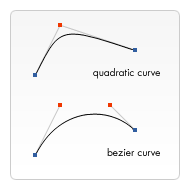# 使用 canvas 来绘制图形

## 栅格## 绘制矩形

`fillRect(x, y, width, height)`

`strokeRect(x, y, width, height)`

`clearRect(x, y, width, height)`

### 矩形（Rectangular）例子

js

``````function draw() {
var canvas = document.getElementById("canvas");
if (canvas.getContext) {
var ctx = canvas.getContext("2d");

ctx.fillRect(25, 25, 100, 100);
ctx.clearRect(45, 45, 60, 60);
ctx.strokeRect(50, 50, 50, 50);
}
}
``````

`fillRect()`函数绘制了一个边长为 100px 的黑色正方形。`clearRect()`函数从正方形的中心开始擦除了一个 60*60px 的正方形，接着`strokeRect()`在清除区域内生成一个 50*50 的正方形边框。

## 绘制路径

1. 首先，你需要创建路径起始点。
2. 然后你使用画图命令去画出路径。
3. 之后你把路径封闭。
4. 一旦路径生成，你就能通过描边或填充路径区域来渲染图形。

`beginPath()`

`closePath()`

`stroke()`

`fill()`

### 绘制一个三角形

js

``````function draw() {
var canvas = document.getElementById("canvas");
if (canvas.getContext) {
var ctx = canvas.getContext("2d");

ctx.beginPath();
ctx.moveTo(75, 50);
ctx.lineTo(100, 75);
ctx.lineTo(100, 25);
ctx.fill();
}
}
``````

### 移动笔触

`moveTo(x, y)`

js

``````function draw() {
var canvas = document.getElementById("canvas");
if (canvas.getContext) {
var ctx = canvas.getContext("2d");

ctx.beginPath();
ctx.arc(75, 75, 50, 0, Math.PI * 2, true); // 绘制
ctx.moveTo(110, 75);
ctx.arc(75, 75, 35, 0, Math.PI, false); // 口 (顺时针)
ctx.moveTo(65, 65);
ctx.arc(60, 65, 5, 0, Math.PI * 2, true); // 左眼
ctx.moveTo(95, 65);
ctx.arc(90, 65, 5, 0, Math.PI * 2, true); // 右眼
ctx.stroke();
}
}
``````

### 线

`lineTo(x, y)`

js

``````function draw() {
var canvas = document.getElementById("canvas");
if (canvas.getContext) {
var ctx = canvas.getContext("2d");

// 填充三角形
ctx.beginPath();
ctx.moveTo(25, 25);
ctx.lineTo(105, 25);
ctx.lineTo(25, 105);
ctx.fill();

// 描边三角形
ctx.beginPath();
ctx.moveTo(125, 125);
ctx.lineTo(125, 45);
ctx.lineTo(45, 125);
ctx.closePath();
ctx.stroke();
}
}
``````

### 圆弧

`arc(x, y, radius, startAngle, endAngle, anticlockwise)`

`arcTo(x1, y1, x2, y2, radius)`

x,y 坐标是可变的。半径（radius）和开始角度（startAngle）都是固定的。结束角度（endAngle）在第一列开始时是 180 度（半圆）然后每列增加 90 度。最后一列形成一个完整的圆。

`clockwise`语句作用于第一、三行是顺时针的圆弧，`anticlockwise`作用于二、四行为逆时针圆弧。`if`语句让一、二行描边圆弧，下面两行填充路径。

js

``````function draw() {
var canvas = document.getElementById("canvas");
if (canvas.getContext) {
var ctx = canvas.getContext("2d");

for (var i = 0; i < 4; i++) {
for (var j = 0; j < 3; j++) {
ctx.beginPath();
var x = 25 + j * 50; // x 坐标值
var y = 25 + i * 50; // y 坐标值
var radius = 20; // 圆弧半径
var startAngle = 0; // 开始点
var endAngle = Math.PI + (Math.PI * j) / 2; // 结束点
var anticlockwise = i % 2 == 0 ? false : true; // 顺时针或逆时针

ctx.arc(x, y, radius, startAngle, endAngle, anticlockwise);

if (i > 1) {
ctx.fill();
} else {
ctx.stroke();
}
}
}
}
}
``````

### 二次贝塞尔曲线及三次贝塞尔曲线

`quadraticCurveTo(cp1x, cp1y, x, y)`

`bezierCurveTo(cp1x, cp1y, cp2x, cp2y, x, y)`右边的图能够很好的描述两者的关系，二次贝塞尔曲线有一个开始点（蓝色）、一个结束点（蓝色）以及一个控制点（红色），而三次贝塞尔曲线有两个控制点。

#### 二次贝塞尔曲线

js

``````function draw() {
var canvas = document.getElementById("canvas");
if (canvas.getContext) {
var ctx = canvas.getContext("2d");

// 二次贝塞尔曲线
ctx.beginPath();
ctx.moveTo(75, 25);
ctx.stroke();
}
}
``````

#### 三次贝塞尔曲线

js

``````function draw() {
var canvas = document.getElementById("canvas");
if (canvas.getContext) {
var ctx = canvas.getContext("2d");

//三次贝塞尔曲线
ctx.beginPath();
ctx.moveTo(75, 40);
ctx.bezierCurveTo(75, 37, 70, 25, 50, 25);
ctx.bezierCurveTo(20, 25, 20, 62.5, 20, 62.5);
ctx.bezierCurveTo(20, 80, 40, 102, 75, 120);
ctx.bezierCurveTo(110, 102, 130, 80, 130, 62.5);
ctx.bezierCurveTo(130, 62.5, 130, 25, 100, 25);
ctx.bezierCurveTo(85, 25, 75, 37, 75, 40);
ctx.fill();
}
}
``````

### 矩形

`rect(x, y, width, height)`

### 组合使用

js

``````function draw() {
var canvas = document.getElementById("canvas");
if (canvas.getContext) {
var ctx = canvas.getContext("2d");

roundedRect(ctx, 12, 12, 150, 150, 15);
roundedRect(ctx, 19, 19, 150, 150, 9);
roundedRect(ctx, 53, 53, 49, 33, 10);
roundedRect(ctx, 53, 119, 49, 16, 6);
roundedRect(ctx, 135, 53, 49, 33, 10);
roundedRect(ctx, 135, 119, 25, 49, 10);

ctx.beginPath();
ctx.arc(37, 37, 13, Math.PI / 7, -Math.PI / 7, false);
ctx.lineTo(31, 37);
ctx.fill();

for (var i = 0; i < 8; i++) {
ctx.fillRect(51 + i * 16, 35, 4, 4);
}

for (i = 0; i < 6; i++) {
ctx.fillRect(115, 51 + i * 16, 4, 4);
}

for (i = 0; i < 8; i++) {
ctx.fillRect(51 + i * 16, 99, 4, 4);
}

ctx.beginPath();
ctx.moveTo(83, 116);
ctx.lineTo(83, 102);
ctx.bezierCurveTo(83, 94, 89, 88, 97, 88);
ctx.bezierCurveTo(105, 88, 111, 94, 111, 102);
ctx.lineTo(111, 116);
ctx.lineTo(106.333, 111.333);
ctx.lineTo(101.666, 116);
ctx.lineTo(97, 111.333);
ctx.lineTo(92.333, 116);
ctx.lineTo(87.666, 111.333);
ctx.lineTo(83, 116);
ctx.fill();

ctx.fillStyle = "white";
ctx.beginPath();
ctx.moveTo(91, 96);
ctx.bezierCurveTo(88, 96, 87, 99, 87, 101);
ctx.bezierCurveTo(87, 103, 88, 106, 91, 106);
ctx.bezierCurveTo(94, 106, 95, 103, 95, 101);
ctx.bezierCurveTo(95, 99, 94, 96, 91, 96);
ctx.moveTo(103, 96);
ctx.bezierCurveTo(100, 96, 99, 99, 99, 101);
ctx.bezierCurveTo(99, 103, 100, 106, 103, 106);
ctx.bezierCurveTo(106, 106, 107, 103, 107, 101);
ctx.bezierCurveTo(107, 99, 106, 96, 103, 96);
ctx.fill();

ctx.fillStyle = "black";
ctx.beginPath();
ctx.arc(101, 102, 2, 0, Math.PI * 2, true);
ctx.fill();

ctx.beginPath();
ctx.arc(89, 102, 2, 0, Math.PI * 2, true);
ctx.fill();
}
}

// 封装的一个用于绘制圆角矩形的函数。

function roundedRect(ctx, x, y, width, height, radius) {
ctx.beginPath();
ctx.lineTo(x, y + height - radius);
ctx.lineTo(x + width - radius, y + height);
ctx.quadraticCurveTo(x + width, y + height, x + width, y + height - radius);
ctx.lineTo(x + width, y + radius);
ctx.stroke();
}
``````

## Path2D 对象

`Path2D()`

`Path2D()`会返回一个新初始化的 Path2D 对象（可能将某一个路径作为变量——创建一个它的副本，或者将一个包含 SVG path 数据的字符串作为变量）。

js

``````new Path2D(); // 空的 Path 对象
new Path2D(path); // 克隆 Path 对象
new Path2D(d); // 从 SVG 建立 Path 对象
``````

Path2D API 添加了 `addPath`作为将`path`结合起来的方法。当你想要从几个元素中来创建对象时，这将会很实用。比如：

`Path2D.addPath(path [, transform])`

### Path2D 示例

js

``````function draw() {
var canvas = document.getElementById("canvas");
if (canvas.getContext) {
var ctx = canvas.getContext("2d");

var rectangle = new Path2D();
rectangle.rect(10, 10, 50, 50);

var circle = new Path2D();
circle.moveTo(125, 35);
circle.arc(100, 35, 25, 0, 2 * Math.PI);

ctx.stroke(rectangle);
ctx.fill(circle);
}
}
``````

### 使用 SVG paths

js

``````var p = new Path2D("M10 10 h 80 v 80 h -80 Z");
``````